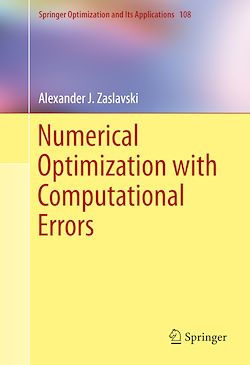# Numerical Optimization with Computational Errors

-English
304 Pages

Description

This book studies the approximate solutions of optimization problems in the presence of computational errors. A number of results are presented on the convergence behavior of algorithms in a Hilbert space; these algorithms are examined taking into account computational errors. The author illustrates that algorithms generate a good approximate solution, if computational errors are bounded from above by a small positive constant. Known computational errors  are examined with the aim of determining an approximate solution. Researchers and students interested in the optimization theory and its applications will find this book instructive and informative.

This monograph contains 16 chapters; including a chapters devoted to the subgradient projection algorithm, the mirror descent algorithm, gradient projection algorithm, the Weiszfelds method, constrained convex minimization problems, the convergence of a proximal point method in a Hilbert space, the continuous subgradient method, penalty methods and Newton’s method.

Subjects

##### Calculus

Informations